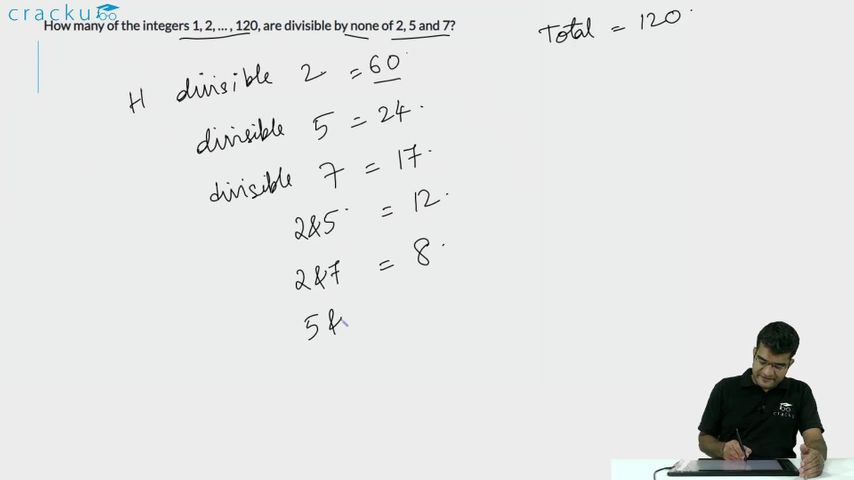Question 69

# How many of the integers 1, 2, … , 120, are divisible by none of 2, 5 and 7?

Solution

The number of multiples of 2 between 1 and 120 = 60

The number of multiples of 5 between 1 and 120 which are not multiples of 2 = 12

The number of multiples of 7 between 1 and 120 which are not multiples of 2 and 5 = 7

Hence, number of the integers 1, 2, … , 120, are divisible by none of 2, 5 and 7 = 120 - 60 - 12 - 7 = 41

### View Video Solution